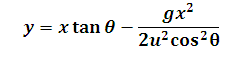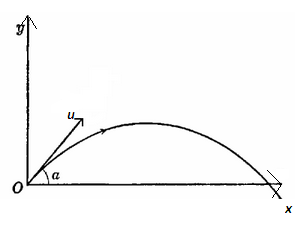# Projectile Motion Trajectory Calculator

This Calctown Calculator calculates the trajectory of a projectile motion.

m
deg
m/s

#### Result

mClick here to view image

where

y = vertical displacement from point of projection

x = horizontal displacement from point of projection

θ = angle of projection

u = velocity of projection

g = acceleration due to gravity